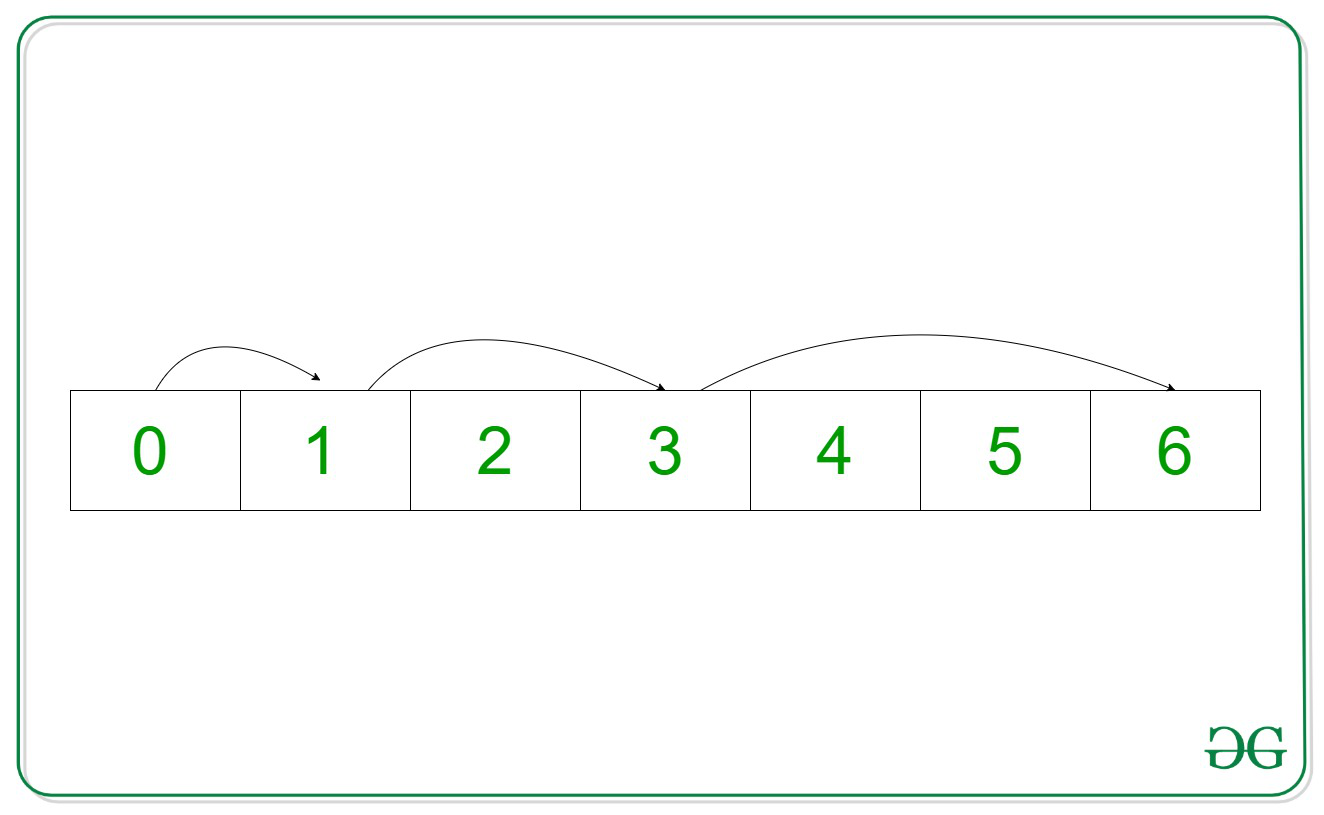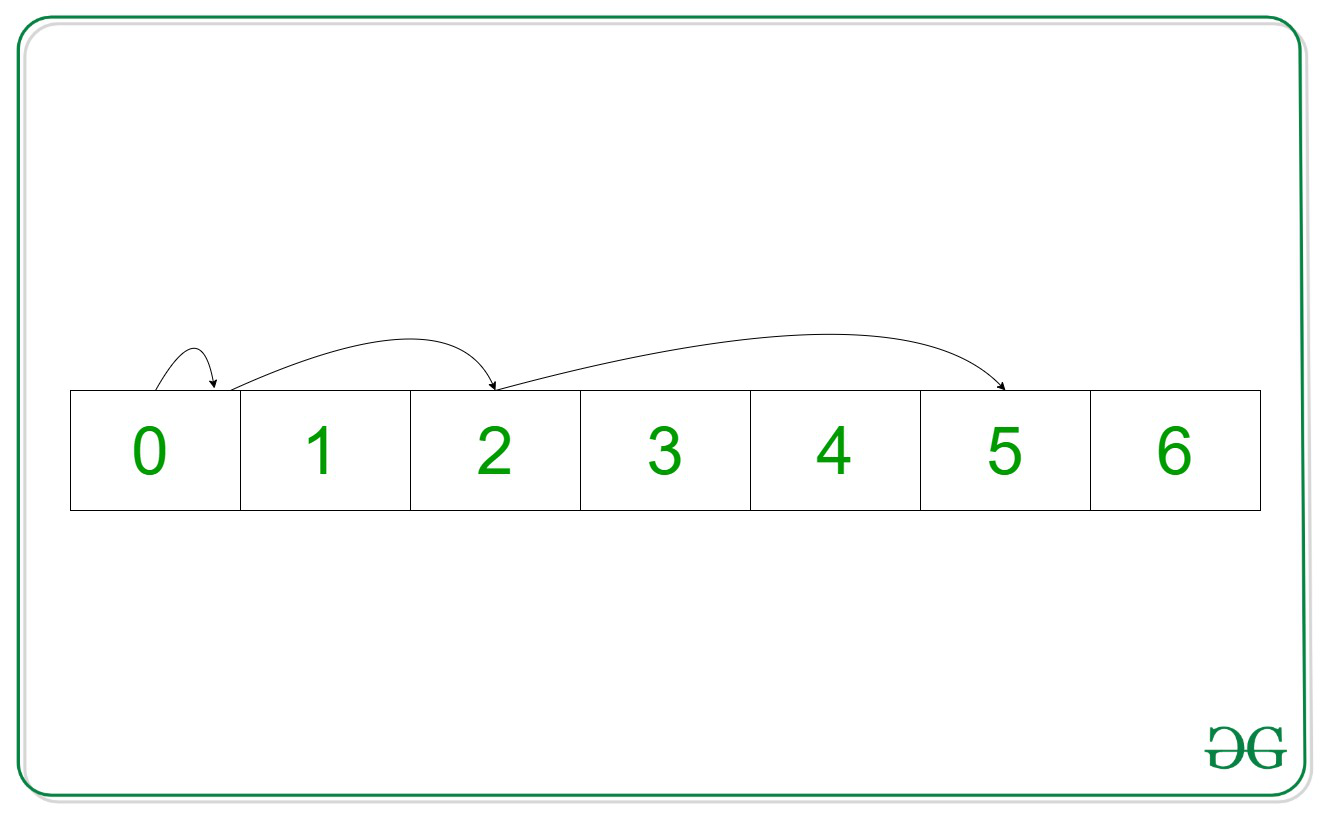# Maximum index a pointer can reach in N steps by avoiding a given index B

• Difficulty Level : Medium
• Last Updated : 09 Apr, 2021

Given two integers N and B, the task is to print the maximum index a pointer, starting from 0th index can reach in an array of natural numbers(i.e., 0, 1, 2, 3, 4, 5…), say arr[], in N steps without placing itself at index B at any point.

In each step, the pointer can move from the Current Index to a Jumping Index or can remain at the Current Index.
Jumping Index = Current Index + Step Number

Examples:

Input: N = 3, B = 2
Output: 6
Explanation:Step 1:
Current Index = 0
Step Number = 1
Jumping Index = 0 + 1 = 1
Step 2:Current Index = 1
Step Number = 2
Jumping Index = 1 + 2 = 3
Step 3:
Current Index = 3
Step Number = 3
Jumping Index = 3 + 3 = 6
Therefore, the maximum index that can be reached is 6.

Input: N = 3, B = 1
Output: 5
Explanation:Step 1:
Current Index = 0
Step Number = 1
Jumping Index = 0 + 1 = 1But this is bad index. So pointer remains at the Current Index.
Step 2:
Current Index = 0
Step Number = 2
Jumping Index = 0 + 2 = 2
Step 3:
Current Index = 2
Step Number = 3
Jumping Index = 2 + 3 = 5
Therefore, the maximum index that can be reached is 5.

Naive Approach: The simplest approach to solve the problem is to calculate the maximum index by considering two possibilities for every Current Index, either to move the pointer by Step Number or by remaining at the Current Index, and generate all possible combinations. Finally, print the maximum index obtained.

Time Complexity: O(N3)
Auxiliary Space: O(1)

Efficient Approach:
Calculate the maximum index that can be reached within the given steps. If the 0th Index can be reached from the maximum index by avoiding the bad index, print the result. Otherwise, repeat the procedure by decrementing the maximum index by 1.
Below are the steps:

1. Calculate the maximum index that can be reached in N steps by calculating the sum of the first N natural numbers.
2. Assign the value of the calculated maximum index to the Current Index.
3. Keep decrementing Current Index by Step Number and Step Number by 1 until one of them becomes negative.
4. After every decrement, check if the Current Index is equal to B or not. If found to be true, revert the changes made on the Current Index.
5. If the Current Index reaches 0 successfully, print the current value of the maximum index as the answer.
6. Otherwise, decrement the value of the maximum index by 1 and repeat from step 2.

Below is the implementation of the above approach:

## C++

 `// C++ program for the above approach``#include ``using` `namespace` `std;` `// Function to find the maximum``// index the pointer can reach``void` `maximumIndex(``int` `N, ``int` `B)``{``    ``int` `max_index = 0;` `    ``// Calculate maximum possible``    ``// index that can be reached``    ``for` `(``int` `i = 1; i <= N; i++) {` `        ``max_index += i;``    ``}` `    ``int` `current_index = max_index, step = N;` `    ``while` `(1) {` `        ``// Check if current index and step``        ``// both are greater than 0 or not``        ``while` `(current_index > 0 && N > 0) {` `            ``// Decrement current_index by step``            ``current_index -= N;` `            ``// Check if current index is``            ``// equal to B or not``            ``if` `(current_index == B) {` `                ``// Restore to previous index``                ``current_index += N;``            ``}` `            ``// Decrement step by one``            ``N--;``        ``}` `        ``// If it reaches the 0th index``        ``if` `(current_index <= 0) {` `            ``// Print result``            ``cout << max_index << endl;``            ``break``;``        ``}` `        ``// If max index fails to``        ``// reach the 0th index``        ``else` `{` `            ``N = step;` `            ``// Store max_index - 1 in current index``            ``current_index = max_index - 1;` `            ``// Decrement max index``            ``max_index--;` `            ``// If current index is equal to B``            ``if` `(current_index == B) {` `                ``current_index = max_index - 1;` `                    ``// Decrement current index``                    ``max_index--;``            ``}``        ``}``    ``}``}` `// Driver Code``int` `main()``{``    ``int` `N = 3, B = 2;``    ``maximumIndex(N, B);``    ``return` `0;``}`

## Java

 `// Java program for``// the above approach``import` `java.util.*;``class` `GFG{` `// Function to find the maximum``// index the pointer can reach``static` `void` `maximumIndex(``int` `N,``                        ``int` `B)``{``int` `max_index = ``0``;` `// Calculate maximum possible``// index that can be reached``for` `(``int` `i = ``1``; i <= N; i++)``{``    ``max_index += i;``}` `int` `current_index = max_index,``                    ``step = N;` `while` `(``true``)``{``    ``// Check if current index``    ``// and step both are greater``    ``// than 0 or not``    ``while` `(current_index > ``0` `&&``        ``N > ``0``)``    ``{``    ``// Decrement current_index``    ``// by step``    ``current_index -= N;` `    ``// Check if current index``    ``// is equal to B or not``    ``if` `(current_index == B)``    ``{``        ``// Restore to previous``        ``// index``        ``current_index += N;``    ``}` `    ``// Decrement step by one``    ``N--;``    ``}` `    ``// If it reaches the 0th index``    ``if` `(current_index <= ``0``)``    ``{``    ``// Print result``    ``System.out.print(max_index + ``"\n"``);``    ``break``;``    ``}` `    ``// If max index fails to``    ``// reach the 0th index``    ``else``    ``{``    ``N = step;` `    ``// Store max_index - 1 in``    ``// current index``    ``current_index = max_index - ``1``;` `    ``// Decrement max index``    ``max_index--;` `    ``// If current index is``    ``// equal to B``    ``if` `(current_index == B)``    ``{``        ``current_index = max_index - ``1``;` `        ``// Decrement current index``        ``max_index--;``    ``}``    ``}``}``}` `// Driver Code``public` `static` `void` `main(String[] args)``{``int` `N = ``3``, B = ``2``;``maximumIndex(N, B);``}``}` `// This code is contributed by gauravrajput1`

## Python3

 `# Python3 program for the above approach` `# Function to find the maximum``# index the pointer can reach``def` `maximumIndex(N, B):``    ` `    ``max_index ``=` `0` `    ``# Calculate maximum possible``    ``# index that can be reached``    ``for` `i ``in` `range``(``1``, N ``+` `1``):``        ``max_index ``+``=` `i` `    ``current_index ``=` `max_index``    ``step ``=` `N` `    ``while` `(``1``):` `        ``# Check if current index and step``        ``# both are greater than 0 or not``        ``while` `(current_index > ``0` `and` `N > ``0``):` `            ``# Decrement current_index by step``            ``current_index ``-``=` `N` `            ``# Check if current index is``            ``# equal to B or not``            ``if` `(current_index ``=``=` `B):` `                ``# Restore to previous index``                ``current_index ``+``=` `N` `            ``# Decrement step by one``            ``N ``-``=` `1` `        ``# If it reaches the 0th index``        ``if` `(current_index <``=` `0``):``            ` `            ``# Print result``            ``print``(max_index)``            ``break` `        ``# If max index fails to``        ``# reach the 0th index``        ``else``:``            ``N ``=` `step` `            ``# Store max_index - 1 in current index``            ``current_index ``=` `max_index ``-` `1` `            ``# Decrement max index``            ``max_index ``-``=` `1` `            ``# If current index is equal to B``            ``if` `(current_index ``=``=` `B):``                ``current_index ``=` `max_index ``-` `1` `                ``# Decrement current index``                ``max_index ``-``=` `1` `# Driver Code``if` `__name__ ``=``=` `'__main__'``:``    ` `    ``N ``=` `3``    ``B ``=` `2``    ` `    ``maximumIndex(N, B)` `# This code is contributed by mohit kumar 29`

## C#

 `// C# program for the``// above approach``using` `System;`` ` `class` `GFG{``    ` `// Function to find the maximum``// index the pointer can reach``static` `void` `maximumIndex(``int` `N,``                         ``int` `B)``{``  ``int` `max_index = 0;`` ` `  ``// Calculate maximum possible``  ``// index that can be reached``  ``for``(``int` `i = 1; i <= N; i++)``  ``{``    ``max_index += i;``  ``}`` ` `  ``int` `current_index = max_index,``                      ``step = N;`` ` `  ``while` `(``true``)``  ``{``      ` `    ``// Check if current index``    ``// and step both are greater``    ``// than 0 or not``    ``while` `(current_index > 0 &&``                       ``N > 0)``    ``{``      ` `      ``// Decrement current_index``      ``// by step``      ``current_index -= N;`` ` `      ``// Check if current index``      ``// is equal to B or not``      ``if` `(current_index == B)``      ``{``          ` `        ``// Restore to previous``        ``// index``        ``current_index += N;``      ``}`` ` `      ``// Decrement step by one``      ``N--;``    ``}`` ` `    ``// If it reaches the 0th index``    ``if` `(current_index <= 0)``    ``{``        ` `      ``// Print result``      ``Console.Write(max_index + ``" "``);``      ``break``;``    ``}`` ` `    ``// If max index fails to``    ``// reach the 0th index``    ``else``    ``{``      ``N = step;`` ` `      ``// Store max_index - 1 in``      ``// current index``      ``current_index = max_index - 1;`` ` `      ``// Decrement max index``      ``max_index--;`` ` `      ``// If current index is``      ``// equal to B``      ``if` `(current_index == B)``      ``{``          ` `        ``current_index = max_index - 1;`` ` `        ``// Decrement current index``        ``max_index--;``      ``}``    ``}``  ``}``}` `// Driver code``public` `static` `void` `Main (String[] args)``{``  ``int` `N = 3, B = 2;``  ` `  ``maximumIndex(N, B);``}``}` `// This code is contributed by offbeat`

## Javascript

 ``
Output:
`6`

Time Complexity: O(N2)
Auxiliary Space: O(1)

My Personal Notes arrow_drop_up